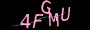×

# 谈谈项目中几个经典的jquery定时器问题`setInterval("fn()",2000);`

```\$(function(){
setInterval("fn()",2000);
})```

```\$(function(){
setInterval(fn,2000);
})```

```\$(function(){
\$.extend({
fn:function(){
}
});
setInterval("\$.fn()",2000);
});```

```\$(function(){
setInterval(fn(para),2000);
})```

```\$(function(){
setInterval(function(){fn(para)},2000);
})```

OK，发现报错已经解除。

```\$(function(){
\$start = 1;
\$.extend({
a:function(t){
\$index = t;
\$start++;
}
});
setInterval("\$.a("+\$start+")",2000);
});```

setInterval("\$.a(1)",2000); 那么结果也就无可厚非了。好吧，只能继续优化下代码：

```\$(function(){
\$start = 1;
\$.extend({
a:function(t){
\$index = t;
\$start++;
}
});
setInterval("\$.a(\$start)",2000);
});```

### 总结

https://www.jiangweishan.com/article/jQuerydingshiqi.html

### 网友评论文明上网理性发言已有0人参与